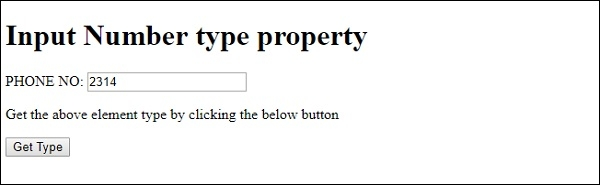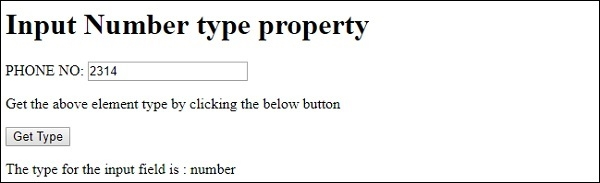# HTML DOM Input Number type Property

The HTML DOM Input number type property is associated with the input element having its type=”number”. It will always return number for the input number element.

## Syntax

Following is the syntax for number type property −

numberObject.type

## Example

Let us look at an example for the HTML DOM Input Number type property −

<!DOCTYPE html>
<html>
<body>
<h1>Input Number type property</h1>
PHONE NO: <input type="number" id="NUMBER1">
<p>Get the above element type by clicking the below button</p>
<button onclick="getType()">Get Type</button>
<p id="Sample"></p>
<script>
function getType() {
var t = document.getElementById("NUMBER1").type;
document.getElementById("Sample").innerHTML = "The type for the input field is : "+t;
}
</script>
</body>
</html>

## Output

This will produce the following output −On clicking the “Get Type” button −In the above example −

We have created an input field with type number and its id set to “NUMBER1”.

PHONE NO: <input type="number" id="NUMBER1">

We have then created the “Get Type” button that will execute the getType() method when clicked by the user −

<button onclick="getType()">Get Type</button>

The getType() method gets the input element using the getElementById() method and assigns its type attribute value to variable t. This variable is then displayed in the paragraph with id “Sample” using its innerHTML property −

function getType() {
var t = document.getElementById("NUMBER1").type;
document.getElementById("Sample").innerHTML = "The type for the input field is : "+t;
}